# Online Class: Basic Math 101\$55.00
no certificate
- OR -
\$80.00
with CEU Certificate*
Start Right Now!
Taking multiple courses? Save with our platinum program.

• 12
Lessons
• 24
Exams &
Assignments
• 12,418
Students
have taken this course
• 17
Hours
average time
• 1.7
CEUs

## Course Description

Fear fractions no more. And learn whether to add first or multiply first in a string of operations. Whether you are struggling with basic math functions in a course today, you are taking a new job that demands understanding of math, or you simply need to review or remember some part of basic math, this course will bring you up to speed. Learn to really understand addition, subtraction, multiplication, and division; know how to calculate and move among decimals, fractions, and percents; figure out ratios and proportions like a pro; and learn to use a calculator for basic math functions.

This course deals with the nuts and bolts of Math. Students will become equipped with a solid foundation of the mathematical tools required to solve more complex mathematical  operations. This course would benefit any student (regardless of age) who requires help understanding basic math skills in order to pass a placement test, a course, a specific job, or a review or brush up on math skills.

No previous education is required though understanding lesson material assumes students at least have a 7th grade reading level. Lessons will focus on the steps involved in adding, subtracting, multiplying, dividing as well as the order of operations. In addition this course will focus on the various forms of numbers such as fractions, decimals, percents, radical signs, absolute value signs exponents, ratios.
• Completely Online
• Self-Paced
• Printable Lessons
• Full HD Video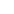• 6 Months to Complete
• Start Anytime
• PC & Mac Compatible
• Android & iOS Friendly
• Accredited CEUs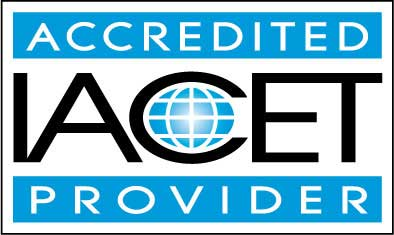## Course Lessons

Average Lesson Rating: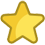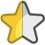#### Lesson 1 : Numbers and Terminology

This lesson focuses on numbers and terminology, such as digits, place values, and variables. 21 Total Points
•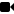Lesson 1 Video A
•Lesson 1 Video B : How to Take an Exam
•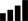Take Poll: Math Phobia
•Take Survey: Reasons for Taking this Course
•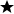Complete: Introduction Assignment
•Complete: Lesson 1 Exercises
•Complete: Quiz for Lesson 1 - Numbers and Terminology

This lesson focuses on addition, both simple and carrying. 20 Total Points
•Lesson 2 Video
•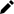Review 9 Practice Worksheets
•Complete: Lesson 2 Exercises
•Complete: Quiz for Lesson 2 - Addition

#### Lesson 3 : Subtraction

This lesson covers subtraction, both basic and borrowing. 20 Total Points
•Lesson 3 Video
•Review 9 Practice Worksheets
•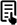Review Article: Basic Subtraction Game
•Complete: Lesson 3 Exercises
•Complete: Quiz for Lesson 3 - Subtraction

#### Lesson 4 : Multiplication

This lesson focuses on multiplication, including both single and multiple digits. 20 Total Points
•Lesson 4 Video
•Review 9 Practice Worksheets
•Review Article: Basic Multiplication Tool
•Complete: Lesson 4 Exercises
•Complete: Quiz for Lesson 4 : Multiplication

#### Lesson 5 - Division

This lesson covers division, including grouping, facts, and single and multiplier divisors. 20 Total Points
•Lesson 5 Video
•Review 10 Practice Worksheets
•Complete: Lesson 5 Exercises
•Complete: Quiz for Lesson 5 - Division

#### Lesson 6 : Signs used in Operations - Positive and Negative Numbers

This lesson focuses on signs used in operations, including positive and negative numbers. 20 Total Points
•Lesson 6 Video
•Review 8 Practice Worksheets
•Take Poll: Positives and Negatives
•Complete: Lesson 6 Exercises
•Complete: Quiz for Lesson 6 - Signs used in Operations – Positive and Negative Numbers

#### Lesson 7 - Operations and Properties

This lesson looks at operations and properties, including closure, and order of operations. 17 Total Points
•Lesson 7 Video
•Review 5 Practice Worksheets
•Complete: Lesson 7 Exercises
•Complete: Quiz for Lesson 7 - Operations and Properties

#### Lesson 8 - Fractions

This lesson focuses on fractions, including adding, subtracting, multiplying, and dividing. 18 Total Points
•Lesson 8 Video
•Review 6 Practice Worksheets
•Review Article: Fraction Video Tutorial
•Complete: Lesson 8 Exercises
•Complete: Quiz for Lesson 8 - Fractions

#### Lesson 9 - Decimals/The Decimal Number System

This lesson focuses on decimals, including moving them, converting fractions, adding and subtracting, etc. 19 Total Points
•Lesson 9 Video
•Review 5 Practice Worksheets
•Complete: Lesson 9 Exercises
•Complete: Quiz for Lesson 9 - Decimals/The Decimal Number System

#### Lesson 10 - Percents

This lesson focuses on using percents, including converting. 20 Total Points
•Lesson 10 Video
•Review 6 Practice Worksheets
•Complete: Lesson 10 Exercises
•Complete: Quiz for Lesson 10 - Percents

#### Lesson 11 - Other Operations

This lesson looks at other operations, including rounding, estimating, exponents, powers, etc. 10 Total Points
•Lesson 11 Video
•Complete: Quiz for Lesson 11 - Other Operations

#### Lesson 12 - Calculators: Using a Calculator

This final lesson focuses on correctly using a calculator. 146 Total Points
•Lesson 12 Video
•Review 5 Practice Worksheets
•Review Article: Review Problems
•Review Video: Tutorial on Using a Calculator
•Take Poll: Using Calculators
•Take Survey: Program Evaluation Follow-up Survey (End of Course)
•Complete: Quiz for Lesson 12 - Calculators : Using a Calculator
•Complete: The Final Exam
351
Total Course Points

## Learning Outcomes

By successfully completing this course, students will be able to:
• Summarize numbers and basic terminology.
• Solve subtraction problems.
• Solve multiplication problems.
• Solve division problems.
• Solve problems involving positive and negative numbers.
• Solve fraction problems.
• Solve decimal problems.
• Solve percent problems, and
• Demonstrate mastery of lesson content at levels of 70% or higher.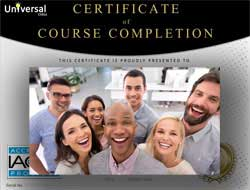• Document Your Lifelong Learning Achievements
• Earn an Official Certificate Documenting Course Hours and CEUs
• Verify Your Certificate with a Unique Serial Number OnlineCourse Title: Basic Math 101
Course Number: 9770448
Course Requirements: View Course Requirements
Lessons Rating: 4.5 / 5 Stars (10,068 votes)
Languages: English - United States, Canada and other English speaking countries
Category:
Course Type: High School Level (Self-Paced, Online Class)
CEU Value: 1.7 IACET CEUs (Continuing Education Units)
CE Accreditation: Universal Class, Inc. has been accredited as an Authorized Provider by the International Association for Continuing Education and Training (IACET).
Assessment Method: Lesson assignments and review exams
Instructor: April Cordry-Moore
Syllabus: View Syllabus
Duration: Continuous: Enroll anytime!
Course Fee: \$55.00 (no CEU Certification) || with Online CEU Certification: \$80.00

Course Only
One Course
No Certificate / No CEUs
\$55
for 6 months

Billed once
This course only
 Includes certificate X Includes CEUs X Self-paced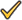Instructor supportTime to complete 6 months No. of courses 1 course
Certificate Course
One Course
Certificate & CEUs
\$80
for 6 months

Billed once
This course only
 Includes certificateIncludes CEUsSelf-pacedInstructor supportTime to complete 6 months No. of courses 1 course
Platinum YearlyALL COURSES
Certificates & CEUs
\$189
per year

Billed once
Includes all 500+ courses
 Includes certificateIncludes CEUsSelf-pacedInstructor supportTime to complete 12 Months No. of courses 500+
Platinum Monthly
ALL COURSES
Certificates & CEUs
\$69 first month
\$29.00 / each consecutive month thereafter

Billed monthly
Includes all 500+ courses
 Includes certificateIncludes CEUsSelf-pacedInstructor supportTime to complete Monthly No. of courses 500+

## Student Testimonials

• "This was a review for me to brush up as well as to get in the swing of practicing Math. I'm preparing for my college Math Proficiency test. This level was fairly easy for me and I'll be taking your Algebra course. I like the video lessons; they were the most helpful. It helps to see the problems illustrated on paper as the instructor explains. This is better than just reading the lesson." -- Arlene H.
• "The videos/lectures/practice problem sets were most helpful." -- Gerardo B.
• "The overall flow of the course and ease in learning was most helpful." -- Shayna S.
• "I enjoyed the class." -- Rubye W.
• "I liked the exercises the lessons had before a quiz. My teacher was great gave me confidence that I could do this and succeed at it." -- Elise E.
• "Loved this class! What was most helpful were the lessons on fractions, decimals, percents - Just getting the basic understanding of how it all works and how they can be converted into one another." -- Gwyneth J.
• "The video instructions were most helpful." -- Jacquelyn K.
• View More Testimonials...